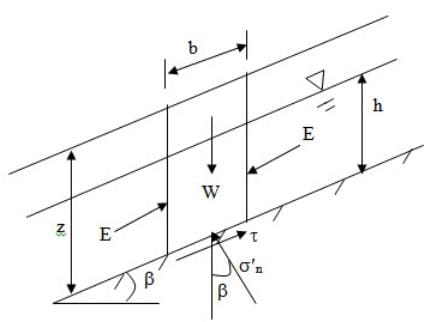Cohesionless & Cohesive Soils - Slope Stability, Soil Mechanics

# Cohesionless & Cohesive Soils - Slope Stability, Soil Mechanics - Notes | Study Soil Mechanics Notes- Agricultural Engineering - Agricultural Engineering

 1 Crore+ students have signed up on EduRev. Have you?

Cohesionless Soil

For cohesionless soil, the shear strength can be written as:

${\tau _f}={\sigma _n}\tan \phi '=\gamma \,z{\cos ^2}\beta \tan \phi '$                        (28.4)

where tf is the failure shear strength and ø' is the angle of shearing resistance or angle of internal friction.

Thus, factor safety (F) can be written as:

$F={{Shear\;Strength} \over {Shear\;Stress}}={{\gamma \,z{{\cos }^2}\beta \tan \phi } \over {\gamma \,z\cos \beta \sin \beta }}={{\tan \phi '} \over {\tan \beta }}$                             (28.5)

Figure 28.3 shows the effective forces acting on an element in the infinite slope. The position of water table is also shown in the figure. The effective normal stress can be written as:

${\sigma '_n}={{(W-{\gamma _w}hb\cos\beta )\cos\beta}\over b}=(\gamma\,z\cos\beta-{\gamma _w}h\cos\beta)\cos\beta$

$=(\gamma \,z - {\gamma _w}h){\cos ^2}\beta$                            (28.6)

$\tau={{W\sin \beta } \over b}=\gamma \,z\cos \beta \sin \beta$                (28.7)

where Yw is the unit weight of water.

$F={{Shear\;Strength}\over{Shear\;Stress}}={{{{\sigma '}_n}\tan \phi}\over\tau }={{(\gamma \,z - {\gamma _w}h){{\cos }^2}\beta\tan\phi}\over{\gamma \,z\cos \beta \sin \beta }}=\left({1 - {{{\gamma _w}h}\over {\gamma \,z}}} \right){{\tan\phi '}\over{\tan\beta}}$               (28.8)

If water table is at the top i.e z=h then,

$F=\left({1 - {{{\gamma _w}} \over \gamma }} \right){{\tan \phi '}\over{\tan \beta }}$                   (28.9)Fig. 28.3. . Effective forces acting on an element in the infinite slope.

Cohesive Soil

In case of c-ø soil, the shear strength can be written as:

${\tau _f}=c' + {\sigma _n}\tan \phi '=c' + \gamma \,z{\cos ^2}\beta \tan \phi '$                                       (28.10)

$F=={{c' + \gamma \,z{{\cos }^2}\beta \tan \phi '} \over {\gamma z\cos \beta \sin \beta }}$                   (28.11)

For F = 1, the depth z is called as critical depth (hc). Thus, putting F=1 and z=hc in Eq. (28.11), one can get

${h_c}={{c'} \over {\gamma (\tan \beta-\tan \phi '){{\cos }^2}\beta }}$                                                  (28.12)

For seepage parallel to the slope,

$F={{c' + (\gamma z - {\gamma _w}h){{\cos }^2}\beta \tan \phi'}\over{\gamma z\cos\beta\sin\beta }}$     (28.13)

${h_c} = \frac{{c'}}{{\gamma (\tan\beta-\frac{{{\gamma _w}}}{\gamma}\tan\varphi '){{\cos}^2}\beta}}$      (28.14)

The stability number (Sn) can be written as:

${S_n}={{c'} \over {\gamma {h_c}}}=(\tan \beta-\tan \phi '){\cos ^2}\beta$

The document Cohesionless & Cohesive Soils - Slope Stability, Soil Mechanics - Notes | Study Soil Mechanics Notes- Agricultural Engineering - Agricultural Engineering is a part of the Agricultural Engineering Course Soil Mechanics Notes- Agricultural Engineering.
All you need of Agricultural Engineering at this link: Agricultural Engineering

## Soil Mechanics Notes- Agricultural Engineering

64 docs
 Use Code STAYHOME200 and get INR 200 additional OFF

## Soil Mechanics Notes- Agricultural Engineering

64 docs

Track your progress, build streaks, highlight & save important lessons and more!

,

,

,

,

,

,

,

,

,

,

,

,

,

,

,

,

,

,

,

,

,

,

,

,

;